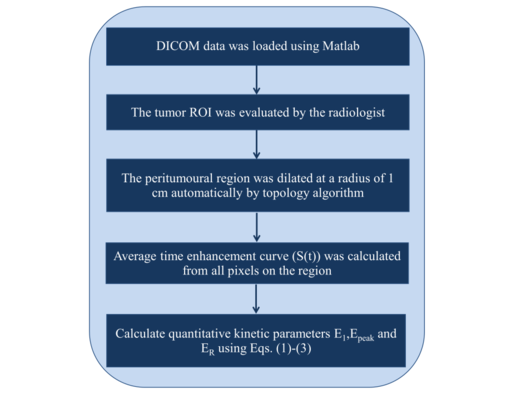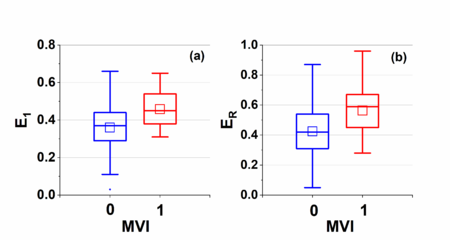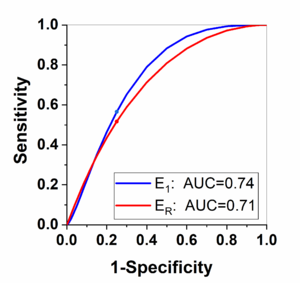## Abstract

The purpose of this study is to predict preoperatively microvascular invasion (MVI) of solitary small hepatocellular cancer (sHCC) by using the kinetic parameters analysis on dynamic enhancement magnetic resonance imaging (MRI). Patients ($n=61$) with known solitary sHCC($\leq 3$cm) were preoperatively examined with Gd-EOB-DTPA-enhanced MRI first before hepatic resection. The arterial peritumoral enhancement measured from the dynamic enhancement-MRI was analyzed by using quantitative kinetic parameters, including initial enhancement ($E_{1}$), peak enhancement ($E_{\rm {peak}}$), and enhancement ratio ($E_{R}$) calculated. Correlations between quantitative kinetic parameters and MVI were evaluated and differences between MVI positive and negative groups were assessed. Histopathological analysis of liver resection confirmed that 19 patients had sHCC with MVI and that 42 patients had sHCC without MVI. Average ($\pm$ standard deviation) $E_{1}$ is $0.36\pm 0.12$ and $0.46\pm 0.09$, $E_{\rm {peak}}$ is $0.78\pm 0.24$ and $0.74\pm 0.18$, and $E_{R}$ is $0.42\pm 0.20$ and $0.56\pm 0.17$ for negative and positive group, respectively. Statistical analysis showed that average $E_{1}$ and ER for the positive group were significantly higher ($p<0.05$) than the negative group. The receiver operating characteristics (ROC) analysis between the two groups had area under the curve of 0.74 and 0.71 for $E_{1}$ and $E_{R}$, respectively. Quantitative kinetic parameters analysis for the arterial peritumoral enhancement is feasibility to the prediction and assist diagnosis of MVI in clinical practice.

Keywords: Microvascular invasion, solitary small hepatocellular cancer, quantitative analysis, kinetic parameters, dynamic enhancement magnetic resonance imaging

## 1. Introduction

Microvessel invasion (MVI) is a major prognostic factor in hepatocellular carcinoma (HCC) that influences the choice of treatment, but rarely can be evaluated preoperatively [1,2]. Several studies have reported that certain imaging findings on dynamic enhancement MRI are useful for predicting MVI of HCC, including tumor size , peritumoral enhancement , tumor margin , tumor hypointensity or peritumoral hypointensity on hepatobiliary phase (HBP) [6,7], radiological capsule on gadoxetic acid–enhanced MR imaging and so on .

Gd-EOB-DTPA enhanced MRI can provide more valuable information for the assessment of HCC and has been widely used in preoperative evaluation settings [9,10]. Some researches use the HBP images to describe the tumor margins in the axial and coronal hepatobiliary phase, including smooth , wedge-shaped peritumoral enhancement  and irregular circumferential peritumoral enhancement .

However, all of these studies are based on qualitative observations and lack the kinetic parameters analysis of arterial phase tumor peritumoral enhancement on MVI. Especially for the small hepatocellular carcinoma (sHCC) with the maximum tumor diameter $\leq 3$cm , there are limited studies that have investigated MR imaging finding for predicting MVI . Therefore, techniques for quantitative analysis of dynamic enhancement MRI should be developed to directly predict MVI of sHCC, which results in early recurrence after hepatic resection and poor prognosis .

To our knowledge this is the first study to quantitatively preoperatively predict solitary sHCC with MVI based on kinetic parameters analysis of dynamic enhancement MRI. The kinetic analysis of pre-enhanced phase, arterial phase (20 seconds), portal venous phase (55 seconds), equilibrium phase (90 seconds) and delayed phase (180 seconds) enhancement curves of dynamic enhancement MRI were used in this study.

## 2. Materials and methods

This study was approved by the Institutional Review Board at our institution and the requirement for informed consent was waived. Patients were recruited from February 2018 to October 2019. The dynamic enhancement MRI was performed within one week before curative hepatectomy, and there is no macroscopic vascular invasion on MRI. A total of 61 patients (average age = 56 years old; 15 females and 46 males) with known single sHCC ($\pm 3$cm) were enrolled in this study. Tumor size, number, and capsule condition were obtained at gross specimen. The entire tumor was examined for sHCC. MVI was defined as the presence of tumor in vascular space of surrounding hepatic tissue line by endothelial cells on microscopy .

MRI examination was performed by using 3.0-T system (Ingenia, Philips Medical Systems, Eindhoven, and The Netherlands) in all patients. 32-channel phased-array coil was used. The scanning scale covered from the top to the lower edge of live. The protocol used consisted of the following sequences which were shown in Table 1.

Table 1. The protocol used consisted of the following sequences
 Sequences Index Value Axial fat-suppressed RT T2W single shot turbo spin echo TR/ TE $535/75$ slice thickness /gap $7/1$ mm FOV $350\times 392$ cm matrix size $232\times 199$ Coronal breath-hold T2W single shot turbo spin echo TR/TE $1100/80$ slice thickness /gap $6/1$ mm FOV $350\times 346$ cm matrix size $292\times 253$ Axial breath-hold dual-echo(in-phase and opposed-phase) T1W fast field-echo TR/TE1/TE2 $106/1.15/2.3$ slice thickness /gap $7/1$ mm FOV $400\times 322$ cm matrix size $244\times 181$ Fat-suppressed dynamic 3D volumetric interpolated breath-hold T1W sequence (before /after injection) TR/TE1/TE2 $3.6/1.32/2.3$ slice thickness /gap $5/-2.5$ mm FOV $320\times 427$ cm matrix size $200\times 250$ Injection of Gd-EOB-DTPA Primovist/ flow rate $0.1$ mL/kg $1$ mL/s saline/ flow rate $20$ml $2$ml/s The dynamic enhanced sequence pre-enhanced phase - arterial phase $20$s after injection portal venous phase $55$ s after injection equilibrium phase $90$s after injection delayed phase $180$s after injection Hepatobiliary phase images - $20$min after injection TR, repetition time; TE, echo time; FOV, field of view; RT, respiratory-triggered

For each patient, the tumor ROI was evaluated by the radiologist (15-year abdominal diagnosis experience). Dynamic enhancement MRI data was analyzed using MATLAB (MathWorks, Natick, MA) with an in-house software package. The peritumoural region (dilated distance was analysed as dilated pixel counts multiplied by pixel size) of the labeled ROI was dilated at a radius of 1 cm automatically by topology algorithm. The peritumoural region in this paper is 8 pixels thick. Then, all the pixels on the peritumoural region were used for kinetic analysis.

ROI Topology Algorithm has follow steps:

Step 1: Search the ROI pixels (value=1);

Step 2: if the product of adjacent pixels value equal 0, that means the pixel is an edge;

Step 3: let the adjacent pixel equal 1 to enlarge the ROI with 1 pixel thick;

So, one loop can make the ROI enlarge 1 pixel thick, and in our research the algorithm need loop 8 times.

For the kinetic analysis, the signal intensity curve ($S(t)$) were utilized from the dynamic enhancement MRI data . For each patient, average peritumoural region enhancement curve was used to calculate the kinetic parameters. The baseline signal intensity value ($S_{0}$) of each pixel was the signal intensity ($S(t)$) of the precontrast time point. Three quantitative kinetic parameters, namely initial enhancement ($E_{1}$), peak enhancement ($E_{\rm {peak}}$), and enhancement ratio ($E_{R}$) were calculated using $S(t)$ as follows :

 $E_{1}=(S_{1}-S_{0}/S_{0}$,
(1)
 $E_{\rm {peak}}=(S_{\rm {peak}}-S_{0})/S_{0}$,
(2)
 $E_{R}=(S_{1}-S_{0})/(S_{\rm {last}}-S_{0})$,
(3)

where $S_{0}$, $S_{1}$, $S_{\rm {peak}}$, and $S_{\rm {last}}$ are the signal intensity at the baseline, the 1st enhancement time point, the peak point and the last time point, respectively. The detailed data analysis steps are shown in Figure 1 flowchart.Figure 1. The flowchart of detailed steps used in the kinetic analysis

Student t-tests were performed to examine whether there were significant differences between MVI positive and negative groups for calculated quantitative parameters. Receiver operating characteristics (ROC) analysis was performed to evaluate whether calculated parameters could be used to distinguish between MVI positive and negative groups. A p-value less than 0.05 was considered as statistically significant.

## 3. Results

### 3.1. Patients

For all 61 patients, they were diagnosed as a MVI positive group ($n=19$) if the invasion of tumor cells within a vascular space lined by endothelium that is visible only on microscopy, and otherwise as an MVI negative group ($n=42$).

Figure 2 shows the peritumoral region and the corresponding signal intensity curve $S(t)$ of a MVI positive patient and a negative one for example. The curve demonstrated that there was clearly higher enhancement for the MVI positive group compared to the MVI negative group.Figure 2. The peritumoural region and the corresponding signal intensity curve $S(t)$ of MVI positive patient, male, 47 years old (a and b) and negative patient, male, 44 years old (c and d). The curve demonstrated that there was clearly faster enhancement (E1) and washout (ER) for the MVI positive group compared to the MVI negative group

### 3.2. Kinetic analysis

Figure 3 shows boxplots of kinetic parameters for both MVI positive and negative groups: (a) initial enhancement $E_{1}$, (b) peak enhancement $E_{\rm {peak}}$, and (c) enhancement ratio $E_{R}$. Average ($\pm SD$) $E_{1}$ is $0.36\pm 0.12$ and $0.46\pm 0.09$, $E_{\rm {peak}}$ is $0.78\pm 0.24$ and $0.74\pm 0.18$, and $E_{R}$ is $0.42\pm 0.20$ and $0.56\pm 0.17$ for negative and positive group, respectively. Statistical analysis showed that average $E_{1}$ and $E_{R}$ for the positive group were significantly higher ($p<0.05$) than the negative group.The $E_{\rm {peak}}$ for positive group was slightly lower ($p=0.49$) than the negative group.Figure 3. Boxplots of parameters for both negative and positive groups: (a) $E_{1}$ and (b) $E_{R}$. The square (□) indicates mean of the data. The $E_{1}$ value for negative group and positive group were $0.36\pm 0.12$ and $0.46\pm 0.09$, the $E_{R}$ value $0.42\pm 0.20$ and $0.56\pm 0.17$ for negative and positive group, respectively. Statistical analysis showed that average $E_{1}$ and $E_{R}$ for the positive group were significantly higher ($p<0.05$) than the negative group

Finally, Figure 4 shows the ROC analysis results for the parameters $E_{1}$ and $E_{R}$ obtained from kinetic analysis parameters. By selecting the Youden index cut-off point, the calculated sensitivity is 0.57 and 0.52; and specificity is 0.75 and 0.75 for the $E_{1}$ and $E_{R}$, respectively. The corresponding cut-off value is 0.44 and 0.58 for the $E_{1}$ and $E_{R}$, respectively.Figure 4. The receiver operating characteristics (ROC) analysis results between MVI negative and MVI positive group for the parameters $E_{1}$ and $E_{R}$. The same color dot on the same color line is indicating the Youden index cut-off point

## 4. Discussion

We investigated the feasibility of using dynamic enhancement MRI to directly assess the peritumoral enhancement for prediction the MVI of sHCC. The dynamic enhancement MRI included pre-enhanced phase, arterial phase, portal venous phase, equilibrium phase and delayed phase, which can help us track the blood supply. The kinetic analysis for dynamic enhancement MRI could be more sensitive to imperceptible changes. Furthermore, the enhancement could be analyzed with the physiological compartment model or mathematical model to obtain the qualitative results of slight changes in the lesion hemodynamics, so as to help doctors make accurate diagnosis. The downside of using enhancement curve is that the dynamic enhancement MRI needs to be acquired with more time points, which may add to the burden of clinical work. However, dynamic enhanced liver examination has become a routine method for clinical diagnosis. We believe that kinetic analysis can bring more reliable information for clinical diagnosis.

Our results demonstrated that the kinetic analysis of peritumoral enhancement measured from the dynamic enhancement MRI could be useful for quantitatively evaluating the MVI of sHCC. The parameters $E_{1}$ and $R$ are higher in the positive group than negative group, which means there are faster blood supply and faster washout in the liver parenchyma around the tumor. The feature indicates there is the same kinetic characteristic in the peritumoral region as intratumoral of HCC which increases the probability of MVI. These agreements were very encouraging to inspire more studies in the future. We believe that this is the first study to quantitatively evaluate peritumoral enhancement measured from the dynamic enhancement MRI for prediction the MVI of sHCC.

Previous studies of predicting the MVI of sHCC were mainly on tumor morphology by evaluating the smooth of tumor margin [6,17], the regularity of tumor shape , the completeness of tumor capsule . There is a lack of relevant quantitative research.

The tumor morphology may be not equal to the MVI of sHCC for several reasons. First, the judgment of sHCC from morphology is limited [20,21]. Second, some image features due to individual blood supply differences may not always appear in the corresponding acquisition phase. Third, these features are mainly evaluated by the naked eye of experienced radiologist. Our quantitative analysis of dynamic enhancement MRI could be used to overcome above problems to some extent by quantifying the prediction the MVI of sHCC.

A potential advantage of dynamic enhancement MRI would be the possibility to perform one-stop examination just before HBP image. As a routine clinical examination, dynamic enhancement data are relatively easy to obtain.

There were several limitations to this study. First, the scale of our research was relatively small, especially the positive group. Second, the study did not include comparisons of blood supply changes between different contrast agents. Third, the sHCC in the study is restricted to diameter not bigger than 3cm. The HCC with diameter between 3 and 5cm should also be predicted in the future. Finally, our developed techniques were not tested on different datasets to evaluate the sensitivity and specificity obtained in this study.

There is potential clinical application using kinetic analysis for dynamic enhancement MRI in assisting the MVI of sHCC. Quantitative analysis parameters could be used as objective measures in diagnosis. Our study would inspire more research on the prediction the MVI for the solitary sHCC patients in the future.

## Funding

This research was funded by Harbin youth reserve talent project (2017RAQXJ102) and Harbin Medical University Cancer Hospitals Haiyan Funds (JJZD2020-17).

## Conflicts of Interest

The authors declare no conflict of interest.

### Document informationPublished on 24/03/20
Accepted on 23/03/20
Submitted on 05/03/20

Volume 36, Issue 1, 2020
DOI: 10.23967/j.rimni.2020.03.003

### Document Score0

Views 17
Recommendations 0